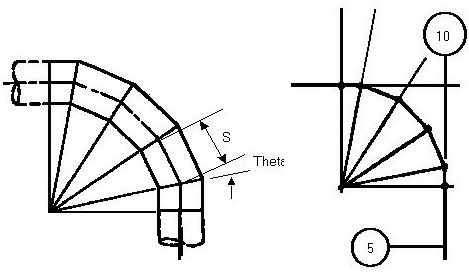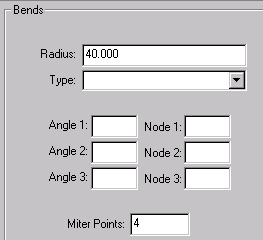# Closely-Spaced Mitered Bend - CAESAR II - Reference Data

## CAESAR II Applications Guide (2019 Service Pack 1)

PPMProduct
CAESAR II
PPMCategory_custom
Reference Data
Version_CAESAR
11.0 (2019)

Miter bends are closely spaced if:

S < r [1 + tan (q)]

Where:

S = miter spacing

r = average pipe cross section radius: (ri+ro)/2

q = one-half the angle between adjacent miter cuts

B > 6 tn

q £ 22.5 deg.

B = length of the miter segment at the crotch.

tn = nominal wall thickness of pipe.

Closely spaced miters, regardless of the number of miter cuts, can be defined as a single bend. CAESAR II always calculates the spacing from the bend radius. If you have the miter spacing but not the bend radius, the radius must be calculated as shown in the following example. The mitered bend shown below has four cuts through 90º and a spacing of 15.913 inches.

 q = a / 2N = 90 / [2(4)] = 11.25º Req = S / [ 2 tan (q)] = 15.913 / [2 tan (11.25º)] = 40